Courses

# Necessary and Sufficient Conditions for Extrema - CSIR-NET Mathematical Sciences Mathematics Notes | EduRev

## Mathematics for IIT JAM, CSIR NET, UGC NET

Created by: Ritwik Kumar

## Mathematics : Necessary and Sufficient Conditions for Extrema - CSIR-NET Mathematical Sciences Mathematics Notes | EduRev

The document Necessary and Sufficient Conditions for Extrema - CSIR-NET Mathematical Sciences Mathematics Notes | EduRev is a part of the Mathematics Course Mathematics for IIT JAM, CSIR NET, UGC NET.
All you need of Mathematics at this link: Mathematics

1 The second variation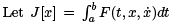be a nonlinear functional, with x(a) = A and x(b) = B ﬁxed. As usual, we will assume that F is as smooth as necessary.
The ﬁrst variation of J is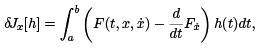where h(t) is assumed as smooth as necessary and in addition satisﬁes h(a) = h(b) = 0. We will call such h admissible.

The idea behind ﬁnding the ﬁrst variation is to capture the linear part of the J [x]. Speciﬁcally, we have

J[x + εh] = J [x] + εδJx[h] + o(ε),

where o(ε) is a quantity that satisﬁes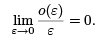The second variation comes out of the quadratic approximation in ε,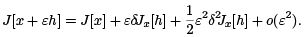It follows that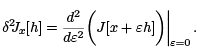To calculate it, we note that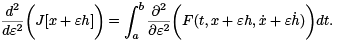Applying the chain rule to the integrand, we see that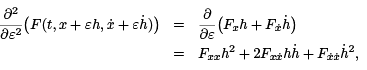where the various derivatives of F are evaluated at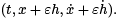Setting ε = 0 and inserting the result in our earlier expression for the second variation, we obtain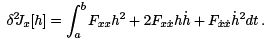Note that the middle term can be written as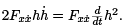Using this in the equation above, integrating by parts, and employing h(a) = h(b) = 0, we arrive at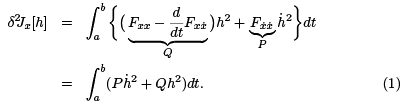2 Legendre’s trick

Ultimately, we are interested in whether a given extremal for J is a weak (relative) minimum or maximum. In the sequel we will always assume that the function x(t) that we are working with is an extremal, so that x(t) satisﬁes the Euler-Lagrange equation,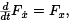makes the ﬁrst variation δJx[h] = 0 for all h, and ﬁxes the functions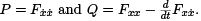To be deﬁnite, we will always assume we are looking for conditions for the extremum to be a weak minimum. The case of a maximum is similar.
Let’s look at the integrand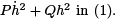It is generally true that a function can be bounded, but have a derivative that varies wildly. Our intuition then says that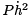is the dominant term, and this turns out to be true. In looking for a minimum, we recall that it is necessary that δ2Jx [h] ≥ 0 for all h. One can use this to show that, for a minimum, it is also necessary, but not suﬃcient, that P ≥ 0 on [a, b]. We will make the stronger assumption that P > 0 on [a, b]. We also assume that P and Q are smooth.

Legendre had the idea to add a term to δ2J to make it nonnegative. Speciﬁcally, he added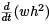to the integrand in (1). Note that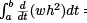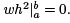Hence, we have this chain of equations,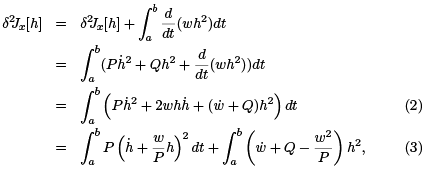where we completed the square to get the last equation. If we can ﬁnd w(t) such that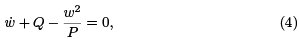then the second variation becomes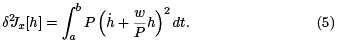Equation (4) is called a Riccati equation. It can be turned into the second order linear ODE below via the substitution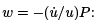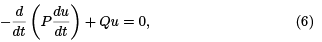which is called the Jacobi equation for J . Two points t = α and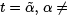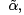are said to be conjugate points for Jacobi’s equation if there is a solution u to (6) such that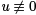between α andand such that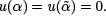When there are no points conjugate to t = a in the interval [a, b], we can construct a solution to (6) that is strictly positive on [a, b]. Start with the two linearly indepemdent solutions u0 and u1 to (6) that satsify the initial conditions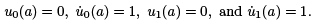Since there is no point in [a, b] conjugate a, u0 (t) ≠ 0 for any a < t ≤ b. In particular, since u˙ 0 (a) = 1 > 0, u(t) will be strictly positive on (a, b]. Next, because u1(a) = 1, there exists t = c, a < c ≤ b, such that u1(t) ≥ 1/2 on [a, c]. Moreover, the continuity of u0 and u1 on [c, b] implies that minc≤t≤b u0(t) = m0 > 0 and minc≤t≤b u1(t) = m1 ∈ R. It is easy to check that on [a, b],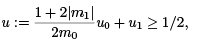and, of course, u solves (6).

This means that the substitutuion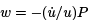yields a solution to the Riccati equation (4), and so the second variation has the form given in (5).
It follows that δ2Jx[h] ≥ 0 for any admissible h. Can the second variation vanish for some h that is nonzero? That is, can we ﬁnd an admissible h ≡ 0 such that δ2Jx[h] = 0? If it did vanish, we would have to have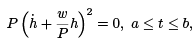and, since P > 0, this implies that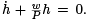This ﬁrst order linear equation has the unique solution,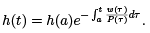However, since h is admissible, h(a) = h(b) = 0, and so h(t) ≡ 0. We have proved the following result.

Proposition 2.1. If there are no points in [a, b] conjugate to t = a, the the second variation is a positive deﬁnite quadratic functional. That is, δ2Jx [h] > 0 for any admissible h not identical ly 0.

3 Conjugate points

There is direct connection between conjugate points and extremals. Let x(t, ε) be a family of extremals for the functional J depending smoothly on a parameter ε. We will assume that x(a, ε) = A, which will be independent of ε. These extremals all satisfy the Euler-Lagrange equation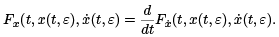If we diﬀerentiate this equation with respect to ε, being careful to correctly apply the chain rule, we obtain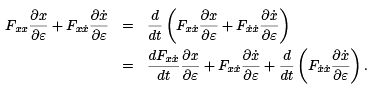Cancelling and rearranging terms, we obtain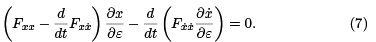Set ε = 0 and let u(t)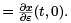Observe that the functions in the equation above, which is called the variational equation, are just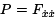and Q =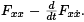Consequently, (7) is simply the Jacobi equation (6). The diﬀerence here is that we always have the initial conditions,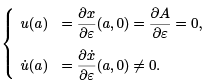We remark that if u˙ (a) = 0, then u(t) ≡ 0.

What do conjugate points mean in this context? Suppose that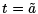is conjugate to t = a. Then we have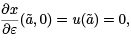which holds independently of how our smooth family of extremals was constructed. It follows that at, we have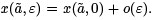. Thus, the family either crosses again at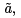or comes close to it, accumulating to order higher than ε there.

4 Suﬃcient conditions

A suﬃcient condition for an extremal to be a relative minimum is that the second variation be strongly positive deﬁnite. This means that there is a c > 0, which is independent of h, such that for all admissible h one has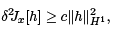where H1 = H1[a, b] denotes the usual Sobolev space of functions with distributional derivatives in L2 [a, b].
Let us return to equation (2), where we added in terms depending on an arbitrary function w. In the integrand there, we will add and subtract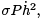where σ is an arbitary constant. The only requirement for now is that 0 < σ < mint∈[a,b] P (t). The result is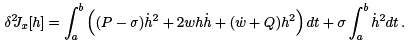For the ﬁrst integral in the term on the right above, we repeat the argument that was used to arrive at (5). Everything is the same, except that P is replaced by P − σ. We arrive at this: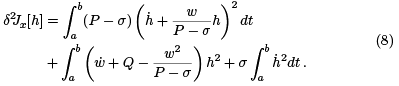We continue as we did in section 2. In the end, we arrive at the new Jacobi equation,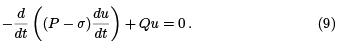The point is that if for the Jacobi equation (6) there are no points in [a, b] conjugate to a, then, because the solutions are continuous functions of the parameter σ, we may choose σ small enough so that for (9) there will be no points conjugate to a in [a, b]. Once we have fouund σ small enough for this to be true, we ﬁx it. We then solve the corresponding Riccati equation and employ it in (8) to obtain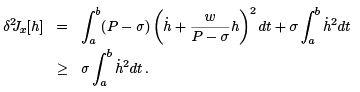Now, for an admissble h, it is easy to show that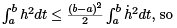that we have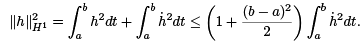Consequently, we obtain this inequality: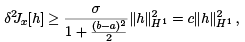which is what we needed for a relative minimum. We summarize what we found below.

Theorem 4.1. A suﬃcient condition for an extremal x(t) to be a relative minimum for the functional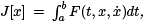where x(a) = A and x(b) = B , is that P (t)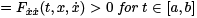and that the interval [a, b] contain no points conjugate to t = a.

Offer running on EduRev: Apply code STAYHOME200 to get INR 200 off on our premium plan EduRev Infinity!

197 docs

,

,

,

,

,

,

,

,

,

,

,

,

,

,

,

,

,

,

,

,

,

;